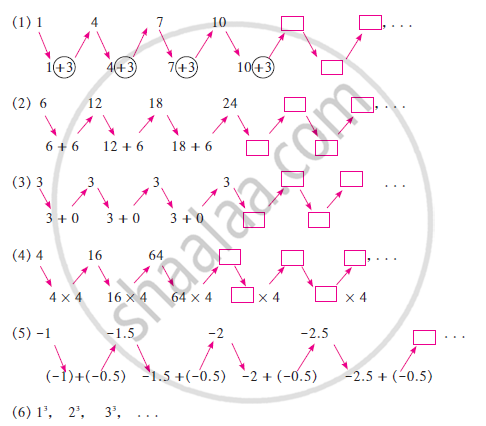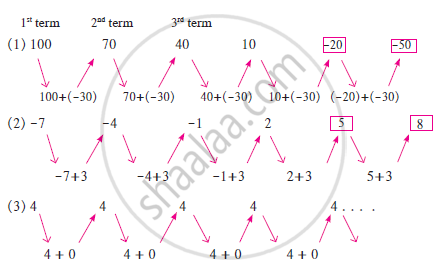Share

# Arithmetic Progression

#### definition

An arithmetic progression is a list of numbers in which each term is obtained by adding a fixed number to the preceding term except the first term.

#### notes

Here in the sequences (1), (2), (3), (5), the similarity is that next term is obtained by adding a particular number to the previous number. Each ot these sequences is called an Arithmetic Progression.Some sequences are given below. For every sequence write the next three terms.
(1) 100, 70, 40, 10, . . . (2) -7, -4, -1, 2, . . . (3) 4, 4, 4, . . .
In the given sequences, observe how the next term is obtained.In each sequence above, every term is obtained by adding a particular number in the previous term. The difference between two consecutive terms is constant. The diference in ex. (i) is negative, in ex. (ii) it is positive and in ex. (iii) it is zero. If the difference between two consecutive terms is constant then it is called the common difference and is generally denoted by letter d.
In the given sequence if the difference between two consecutive terms (tn +1- tn) is constant then the sequence is called Arithmetic Progression (A.P.). In this sequence tn +1- tn = d is the common difference.
In an A.P. if first term is denoted by a and common difference is d then,
t_1 = a , t_2= a + d
t_3= (a + d) + d = a + 2d
A.P. having first term as a and common difference d is
a, (a + d), (a + 2d), (a + 3d), . . . . . .
This is called the general form of an AP.
Some more examples of AP are:
(a) The heights ( in cm ) of some students of a school standing in a queue in the morning assembly are 147 , 148, 149, . . ., 157.
(b) The minimum temperatures ( in degree celsius ) recorded for a week in the month of January in a city, arranged in ascending order are
– 3.1, – 3.0, – 2.9, – 2.8, – 2.7, – 2.6, – 2.5
(c) The balance money ( in ₹ ) after paying 5 % of the total  loan of ₹ 1000 every month is 950, 900, 850, 800, . . ., 50.
(d) The cash prizes ( in ₹ ) given by a school to the toppers of Classes I to XII are, respectively, 200, 250, 300, 350, . . ., 750.
(e) The total savings (in ₹) after every month for 10 months when ₹ 50 are saved each month are 50, 100, 150, 200, 250, 300, 350, 400, 450, 500.
It is left as an exercise for you to explain why each of the lists above is an AP .
Note that in examples (a) to (e) above, there are only a finite number of terms. Such an AP is called a finite AP. Also note that each of these Arithmetic Progressions (APs) has a last term. The APs in examples (i) to (v) in this section, are not finite APs and so they are called infinite Arithmetic Progressions. Such APs do not have a last term.

### Shaalaa.com

Sequences - Recurrence relationships [00:11:36]
S
2
0%

S